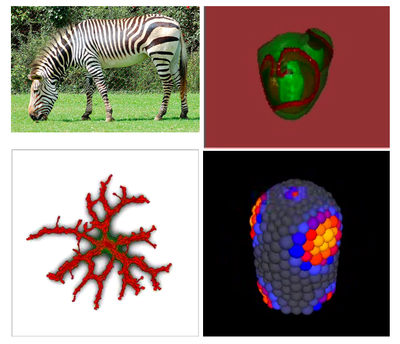### Multiscale Mathematical Biology: from individual cell behavior to biological growth and formIn the autumn semester of 2021, we will be teaching the nineth edition of the course "Multiscale Mathematical Biology" at the Mathematical Institute of Leiden University.

#### Practicalities

The course introduces students to the mathematical and computational biology of multicellular phenomena, covering a range of biological examples, including development of animals and plants, blood vessel growth, bacterial pattern formation and diversification, tumor growth and evolution. The course is also part of the Minor "Quantitative Biology"; students therefore come from a mix of scientific backgrounds, ranging from biology and bioinformatics, to mathematics and physics.  The course consists of a series of lectures,  practical assignments using biological modeling environments, and a final project.

The course will run in the second half of the semester, starting in oktober 2021. The exact format will depend on the circumstances around corona. In 2020 we worked with prerecorded online lectures, followed by physical computer labs. If possible, we will return to a full on-site schedule. Otherwise we will likely combine prerecorded lectures with live-room sessions for discussion. The exact dates for 2021 will be announced in summer 2021.

For homework exercises, in particular the research assignment at the end of the course, it is useful to have Linux installed (Ubuntu) and Qt5.x (see qt.io; select the open source version). Alternatively, we will offer a prepared virtual box (simulated Linux computer running with a program on your PC) with everything installed. If you want to make use of this solution, please install VirtualBox. All software used in the course can be used free of charge.

For more information, see the overview below, or write Roeland an email.

#### Course description:

Biological systems are so complex, that biologists often need to call in the help from mathematicians and computational scientists. These questions constitute a rich source of applied mathematical problems, for which often a range of mathematical and computational techniques need to be combined with one another. Mathematical insight into dynamical systems, pattern formation, complex networks, multiscale dynamics and parallel processing turn out to be a tremendous help while trying to ‘make sense of life’.

This course will in particular introduce you to the mathematical modeling of healthy and diseased multicellular organisms, like ourselves. A key question is how cells cooperate to create biological structure, and how this biological structure feeds back on gene expression. The focus will be on how to sharpen one’s intuition on the emergence of biological systems and patterns by using and further developing a variety of continuous and discrete mathematical models of biological systems.

Mathematical techniques include ordinary-differential equations, partial-differential equations, cellular automata, Hamiltonian systems, and in many cases combinations of those. This course will cover a range of multicellular phenomena, including development of animals and plants, blood vessel networks, bacterial pattern formation and diversification, tumor growth and evolution.

At the end of course students will have an overview of and some hands-on experience with a range of mathematical and computational techniques that computational biologist use in the study of collective cell behavior and biological pattern formation. They are familiar with recent literature on multiscale biological modeling and they have some experience with constructing basic computational models and hypotheses of phenomena described in the biological literature.

#### Overview of topics

• Introduction: role of mathematical modeling in (systems) biology
• Diffusion and transport phenomena, diffusion length, gradients
• Turing patterns; Gierer-Meinhardt equations
• Linear stability analysis
• Biological examples of Turing patterns: hair follicles, skin patterns of zebrafish. So, biology seems to use Turing patterns, with some extra feedback loops here and there. But does it always involve chemical signaling...
• Does biology actually not use Turing patterns? Gap genes in Drosophila
• Introduction to Cellular automata
• Why space matters: understanding growth of tumor spheroids
• Logistic growth; Gompertz law; Eden Growth
• Cellular automata, classes of cellular automata
• Probabilistic cellular automata
• Margolus diffusion in cellular automata - example of Turing patterns using CAs
• Introduction to agent-based and cell-based modeling
• Introduction to the cellular Potts model
• Applications: tumor growth, cell sorting, angiogenesis
• Prebiotic evolution:
• The error threshold
• Hypercycles
• Emergent multi-level selection
• Possibly: genotype-phenotype maps
• cell mechanics
• Other cell-based modeling frameworks:
• Langevin equation
• Vertex-based models
• Dynamics, geometry, and topology of epithelial tissue
• Subcellular-element models
• Lattice-gas cellular automata
• Multiscale Modelling
• Development of Dictyostelium discoideum
• Angiogenesis
• Cancer modeling
##### Computer labs
• Paper & pencil work and numerical simulations - Derive conditions for Turing instability analytically and develop intuition using numerical simulations
• Mutual exclusion of gene expression: modeling the gap gene network in Drosophila melanogaster using the Sharp & Reinitz (1995) model.
• Cellular Automata
• Prebiotic evolution
• Cellular Potts modeling
##### Seminars and mini-projects

In the final part of the course, you will form teams and use the skills you have learned in the course to work on a small research project to follow up on, or inspired by a published paper.

EXAM: T.b.a.

### Paper Presentation Schedule

t.b.a.

##### Practical information:

Course material: Slides will be handed out after the lectures. Reading material: papers. Syllabus in progress.

Material is available at brightspace

Lecturer: Roeland Merks + guest lecturers

Assistants: David Versluis (server is biology.leidenuniv.nl, username is d.m.versluis)

Language: English

Time & location: to be announced,  Leiden University, Snellius (Niels Bohrweg 1)

Methodology: lectures, paper seminar, practical exercises, mini-project, exam.

Required knowledge: Basic background in biology is useful (in particular cell biology or developmental biology) but not required. Some programming skills and familiarity with numerical algorithms and differential equations are useful, but not required.

Evaluation: 1) Practicum assignments; 2) Final project + literature presentation; 3) Written exam

For students of: 3rd Bachelor and 1st Master’s Mathematics and Biology, Minor Quantitative Biology, and other interested students from (Astro)Physics, Pharmaceutical Sciences, and so forth.